Pure and Applied Mathematics Journal
Volume 4, Issue 5-1, October 2015, Pages: 46-50

An Improved Algorithm of Precise Point Cloud Registration

Jun Liu1, 2

1Department of Mathematics and Information Science, Weinan Normal University, Weinan Shaanxi, P. R. China

2Institute of Visualization Technology, Northwest University, Xi’an Shaanxi, P. R. China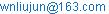Jun Liu. An Improved Algorithm of Precise Point Cloud Registration. Pure and Applied Mathematics Journal. Special Issue: Mathematical Aspects of Engineering Disciplines. Vol. 4, No. 5-1, 2015, pp. 46-50. doi: 10.11648/j.pamj.s.2015040501.19

Abstract: Basing on the application of digital protection of cultural sites, this paper presented a precise algorithm for the multi-resolution point cloud based on sequence iterative. Firstly, based on the synchronous scanning image, a rough registration of the adjacent point cloud can be obtained in an interactive way. Secondly, based on a normal-based algorithm of registration sphere center, the points of sphere surface are filtered from all scanned point cloud data according to the normal data points and the characteristic of viewpoint, and then the center of registration sphere can be calculated accurately and the initial registration of the adjacent point cloud can be obtained by setting Matching Registration Labels mode as the constraint condition. Finally, based on the 3D edge points of the adjacent point cloud from mahalanobis distance calculations, some overlapping images can be eliminated by the way of constantly optimizing the transition matrix in the iteration process. The engineering practice of the Small Wild Goose Pagoda in Tang Dynasty and the Ancient Tomb in Han Dynasty proves that the method is reliable and easy to design and implement and can effectively restrain the accumulative errors of sequence registrations.

Keywords: Digital Archaeology, Multi-View Point Cloud, 3D Registration, Iterative Algorithm

Contents

1. Introduction

It is known to all that cultural sites are the very important material data in research of the ancient history. The point cloud model based on the 3D laser scanning is the foundation to achieve digital protection of the ancient sites. As each scanner can only obtain the partial point cloud data in its position, the multi-view point cloud registration algorithm is required so as to get the complete 3D point cloud model.

The main multi-view point cloud registration methods are ICP algorithm and feature-based registration methods. ICP algorithm assumes that the scene is free of shelters or self-sheltered and the distribution rate of the point cloud samplings are required the same with point as the registration unit. While the initial registration is estimate improperly, the iterative process will fall into local optima (see ). Feature-based registration method does not need the initial registration estimation, but no registration can be obtained without calculation features or with the calculation features insufficient (see ).

In addition to the two main methods, there are some other ones, such as the algorithm proposed Barequet which uses the voting mechanism to achieve partial surface matching; the triangular surface matching method proposed by Arun by using Kalman estimator; the method proposed by Chen which uses the distance between the two surfaces in the normal vector direction to replace the distance from a certain point to its closest point, and takes it as the matching target evaluation function; the method proposed by Masuda which samples point sets at random, and then, with the minimum median squared-error as the measure criterion, re-samples after each iteration; the method proposed by Johnson which uses the featured-extraction strategy to remove the plane points without heuristic information to improve the registration rate; the method proposed in reference which achieves 3D point cloud registration by introducing a reference point; the multi-scanner 3D data fast global registration algorithm of large-scale outdoor scenes based on back projections proposed in reference, which takes the laser sampling points as the registration unit and is suitable for the large-scale site scenes point cloud registration of complex structures; the scattered point cloud data registration algorithm proposed in reference, which, based on the curvature of measured points and normal vector point features, uses the HASH technology to find an effective set of matching points and overcomes the constraints of ICP method which requires more accurate initial position and cannot provide partial matching(see [3,4,5,6,7,8,9,10]).

In 3D scanning process of ancient site scenes, adjacent scanners often need to set different scanning resolutions in order to obtain the details of heritage buildings and relics, which will not ensure the same sampling distribution rates of adjacent point clouds to be registered. Generally, the complex geometrical forms of ancient buildings and relics of the cultural sites make it extremely difficult to calculate their appropriate 3D features from the point cloud data, which means that neither the ICP (Iterative Closest Point) algorithm nor the feature-based registration methods can be applied in the multi-view point cloud registration of cultural site scenes.

In order to decrease the influence of sample resolution, this paper proposes an improved algorithm based on sequence iterative. The algorithm employs two attributes of laser sampling points —position and reflectance to search corresponding points between two overlapping scans by mahalanobis distance, uses back projection to seed up searching corresponding points, and applies interpolation to correcting corresponding points. According to minimal spanning tree, global registration of multiple 3D data sets is completed.

2. Definitions

Definition 1: reflectance image is formed by the reflectivity values of all the sampling points of each view in 3D scenes, and it has all the characteristics of the general images.

Definition 2: The order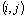of the sampling points and the distance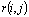from laser the rangefinder to the object generate a 2D image, called range image.

Definition 3: If the 3D point P is given,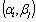can be calculated from equation (1) and then the corresponding pixel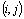can be found in the 2D image, which is the so-called back projection of 3D point P in the 2D range image.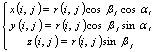(1)

In the equations,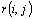is the distance that sampling points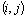correspond from the laser range finder to the object;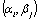are the two azimuth data from horizontal and vertical laser scanning;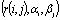and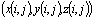are respectively the spherical coordinates and Cartesian coordinates of the scanning points.

If the point setsof the overlapping regions and the corresponding measuring positions of the machine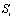and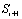are given, the iterative registration procedures are as follows:

1.     In the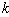iteration, use back projection to determine the two sets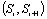of the overlapping area.

2.     Use mahalanobis distance to find the nearest point in the overlap region.

3.     Useand four-element method to recompute the transformation matrix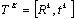, where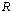is the orthogonal trans-formation matrix and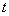is the translation vector.

4.     According to the formula 2, calculate the registration error.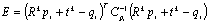(2)

5.     If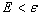, or the number of iterations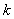is greater than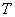, then stop iterating, otherwise go to (1).

6.     Here, the initial transformation of matrix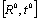is observed by artificial selection of at least 3 same points in the two point sets, and it is obtained by using the formula (3) and the four-element method.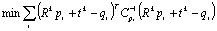(3)

3. Algorithm Rules

3.1. Algorithm Design

Void Seq_Iter_3D_Registraion (Point Cloud initial _Array ], Point Cloud result_Array ])

{s1. Specified registration error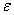and the terminal number of iterations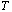.

s2. Calculate coordinates of the calibration sphere center based on the normal vector, so as to realize the initial point cloud registration, and put the results into the array result_Array[ ].

s3. For (i=1; i<=N; i++) // The i is the registration number of scanning station.

{k=1; // The k is the current iteration number.

E=Maximum; //The E is the current registration error.

While (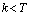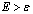)

{

(1)     Determine the overlapping area of the point setsof the adjacent stations.

(2)     The similarity between adjacent reflectance images is calculated based on mahalanobis distance, and the initial coordinate transformation matrix is identified by the sub-image’s centroid of the three pairs of the largest similarity.

(3)     Based on the initial coordinate transformation matrix, the 3D edge points of the adjacent views are translated to the same coordinate system at first, then all of the 3D edge points are registered by using the improved ICP algorithm and the transformation matrix is further optimized.

(4)     Based on the optimized transformation matrix, all of the 3D points of the adjacent views are translated to the same coordinate system.

}}}

3.2. Distance Function Choice

Assume that the scanning point sets of the two adjacent scan stations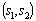are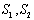. If point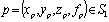, the minimum Euclidean distance of the point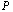to the nearest point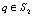is: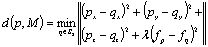(4)

In the equation,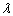is the reflectivity weight. In practice,is difficult to determine due to no unified standard, therefore, the nearest distance and the nearest point will be redefined by using the mahalanobis distance which has statistical properties and invariability while rotating, translating and zooming images.

Definition 4: Assume that point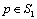is a featured vector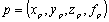and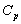is the covariance matrix of point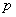and the featured vector of the points near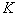, reflecting the distribution of points. The mahalanobis distance of the pointto the nearest point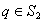is: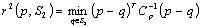(5)

The mahalanobis distance is an effective method to calculate the similarity of two unknown sampling sets and takes into account the links between various features and is independent of scale (Scale-invariant). The distance of the closest point in the mahalanobis distance definition solves the credible problem caused by different variables with different distributions. Its advantages include: (1) under no influence of dimension and with no impact of the distance between two points on the measurement unit of the original data independent; (2) with the same distance between two points calculated from the standardized data and from the centralized data; (3) the distance can also be excluded from the correlation between the disturbance variables, while its disadvantage is that it exaggerates the slight variation of variables.

3.3. Closest Point Determine

Assuming the transformation matrix from point set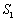to point set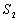is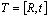,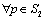is transformed to the coordinate system where the point set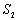is located, and there is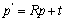. By using the formula (1), calculate the azimuth of point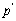related to the scan station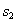, and then determine the scan range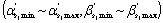from the scanner station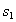to the scanner station, so the overlapping point sets are as:(6)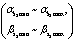is the scanning range of the laser range finder in the scan station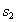and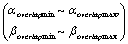is overlapping range of the point setsand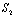.

If point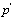is the corresponding point of point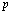converted to the local coordinate system of the scan stationand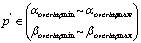, the projection point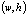is obtained by sending the ray through point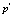from the scanning stationand then through range image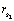. Because of the different resolutions of distance images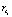and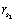from the scanning stationand the scanning station, takewithin point set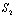as the center and find the closest point of point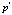in the neighborhood range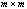, that is,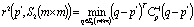(7)

then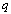is the closest point of point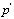.

4. Practice and analysis

This paper discusses the multi-view point cloud data registration of the Small Wild Goose Pagoda tower object with Cyclone 5.5 of the HDS 3000 system as the platform, as shown in Fig. 1. In practice, usually the first or last position of the local scan coordinate system is chosen and the multi-view cloud registration is achieved by the corresponding coordinate transformation.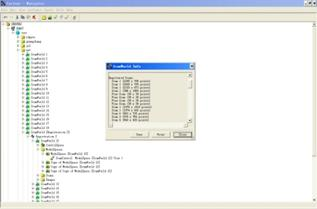Figure 1. HDS3000 Cyclone Platform.

4.1. Registration Process

In practice, we divided the registration process into three stages: rough alignment, initial registration, and registration optimization.

Stage 1 Rough registration manually, with the goal of making the adjacent point cloud roughly aligned. First, select several common points of initial iterative value in two adjacent point clouds by using human-computer interaction, so that the initial iterative value can be as close to the true values as possible. This can not only avoid the divergent phenomena from ICP algorithm but also speed up the convergence rate and reduce the computing time. Second, with the digital photos of the tower body from the synchronal scanning as the reference, set the registration constraint of the adjacent point cloud to "Manually Adding Constraints" mode and adjust the values of constraints continuously to achieve rough registration of the adjacent point cloud of the tower body.

Stage 2 Automatic initial registrations, with the goal of solving the registration error problems in Stage 1. Point cloud by the adjacent alignment between the constraints set to "Matching Registration Labels" model, with calibration object sphere so quickly achieved between adjacent initial point cloud registrations. Registration of this stage is not accurate, for example, there are often some ghosting phenomena, etc. as shown in Fig. 2 (c).

Stage 3 Registration optimization, with the goal of ad-opting the best estimate to make the registration objects arranged as closely as possible in the terminate Scan World. Set the registration constraints of the adjacent point cloud to "Auto-Add Constraints" mode and then observe and assess the registration errors. If the result is not satisfactory, cancel the registration operation. Add one or two constraints and make more registrations to obtain satisfactory results, as shown in Fig. 2 (d).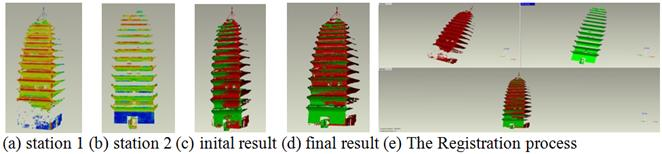Figure 2. Registration Process of the multi-view point cloud by using Sequence Iterative Method.

4.2. Results Analysis

As to the massive data in the point cloud registration of large site scenes, there are two critical problems: (1) how to avoid the iterative divergences; and (2) how to eliminate ghost images in registration process.

The ICP algorithm searches for the same points in a certain area, so the sum of squares of the minimum distance between the same points is also constrained. This will lead to slow point cloud registration and only partial optimum. Especially, the inappropriate choice of initial iterative values will result in very slow convergence rate, even divergent phenomena. Furthermore, the accuracy of calculating the calibration sphere center largely determines the accuracy of the initial registration of multi-view point cloud.

The ancient Chinese architectures and remains are mostly characterized by symmetrical shapes, so the angle of the normals of the plane formed by any three points in the symmetrical parts is less than or even parallel to the critical angle, which will result in relatively large errors of the transformation matrix and even make the selected iterative points far away from the target point in the iteration.

In order to avoid slow convergence or even divergence due to incorrect initial selection, it is advisable to adopt the human-computer interaction to select several common overlapping points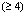in two point cloud as the initial point of iteration process so that initial iterative value is closer to the true value, thereby speeding up the convergence rate and shortening the computing time. Further, by using the normal-based algorithm(see ) for registration sphere center and the normal vector of the scanning data points and view scanning features, we can extract spherical data points of the calibration sphere and accurately calculate the calibration center of the sphere, thereby improving the accuracy of the initial registration. This will not only avoid the iterative divergent phenomena caused by the ICP algorithm and speed up the convergence but also effectively reduce the registration errors. The simulation experiment data (as shown in table 1) verify the correctness and validity of this assumption.

Table 1. The performance comparsion (Unit: [m]).

 Tolerance Iterations Convergence condition Mean residual Standard deviation ICP 4.0 287850 0.000075 0.007576 0.31602 SIM 0.05 42 0.0000089 0.000035 0.011740

Test data set: S1:953257 pixels, S2:559293 pixels

5. Results and Conclusions

As shown in Fig. 3 and Fig. 4, the algorithm proposed in this paper is applied in the projects such as the Small Wild Goose Pagoda in Tang Dynasty and the ZHANG Anshi Ancient Tomb in Han Dynasty. Through calculating accurately the 3D edge point of the adjacent point cloud, optimizing and transforming the matrix in the iterative process, we achieved ideal registration results. The advantages of this method can increase the registration speed by reducing the amount of iterative calculations and can obtain more satisfactory registration results.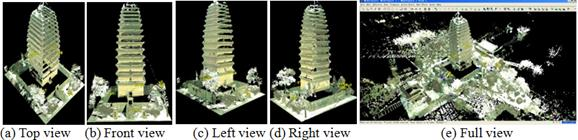Figure 3. Model of the Small Wild Goose Pagoda in Tang Dynasty.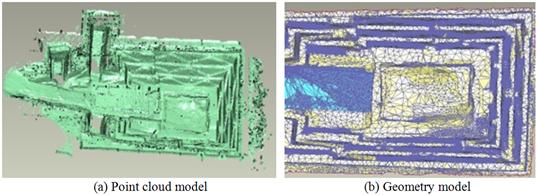Figure 4. Model of the ZHANG An-shi Ancient Tomb in Han Dynasty.

It proves that the method proposed in the paper is simple and efficient, and can inhibit the accumulation of sequence alignment errors. However, the registration process requires more manual operation, so the automatic registration method for multi-view point cloud should be studied in future.

Acknowledgment

The work reported in this paper was founded by the Natural Science Basic Research Plan in Shannxi Province, China (Research and Implementation of Automatic Drawing Methods for Archaeology Drawing based on 3D Model of Cultural Relies, Grant No. 15JK1247).

References

1. P.J. Besl, N.D. McKay, "A method for registration of 3D shapes," IEEE Transactions on Pattern Analysis and Machine Intelligence, 1992, 14(2), pp 239–256.
2. I. Stamos, M. Leordeann,"Automated feature-based range registration of urban scenes of large scale," In: Proceedings of the IEEE Computer Vision and Pattern Recognition, Madison, 2003, 2, pp 18–20.
3. K.S. Arun, T.S. Huang, S.D. Blostein, "Least square fitting of two 3D point sets," IEEE Transactions on Pattern Analysis and Machine Intelligence, 1987, V9(5), pp 698–700.
4. T. Masuda, N. Yokoya, "A robust method for registration and segmentation of multiple range images," Computer Vision and Image Understanding, 1995, V61 (3), pp 295–307.
5. A. Johnson, M. Hebert, "Surface registration by matching oriented points," Proceedings of International Conference on Recent Advances in 3D Digital Imaging and Modeling[C], Ottawa, 1997, pp 121–128.
6. LUO Xianbo, ZHONG Yuexian, LI Renju, "Data registration in 3-D scanning systems," Tsinghua Univ (Sci &Tech), 2004, V44 (8), pp 1104–1106.
7. Zhang Aiwa, Sun Weidong, Ge Chenghui, "Fast Gobal Registration of Multiple 3D Data Sets from Outdoor Large Scenes," High Technology Letters, 2004, V14(6), pp 6–13.
8. Zhu Yanjuan, Zhou Laishui, Zhang Liyan, "Registration of Scattered Cloud Data," Journal of Computer-adiedDesign&Computer Graphics, 2006, 18 (4), pp 475–481.
9. Wei Jiang, Xiong Bangshu, Feng Yan, "Normal-based Algorithm for Registration Sphere Center in Multiple Views," Computer Engineering and Applications, 2005, (19), pp 15–17.
10. Lu-shen Wu,Qing-jin Peng,"Research and development of fringe projection-based methods in 3D shape reconstruction,"Journal of Zhejiang University SCIENCE A,2006(6), pp 1026 -1036
11. Dror Aiger,Niloy J. Mitra,Daniel Cohen-Or.,"4-points congruent sets for robust pairwise surface registration,"ACM Transactions on Graphics (TOG),2008 (3).
12. Da Silva J P Jr,Borges D L,de Barros Vidal F., "A dynamic approach for approximate pairwise alignment based on 4-points congruence sets of 3D points,"Proceedings of the 18th IEEE International Conference on Image Processing,2011.
13. Sofien Bouaziz,Andrea Tagliasacchi,Mark Pauly, "Sparse Iterative Closest Point,"Computer Graphics Forum,2013 (5), pp 113–123.

 Contents 1. 2. 3. 3.1. 3.2. 3.3. 4. 4.1. 4.2. 5.
Article ToolsAbstractPDF(336K)